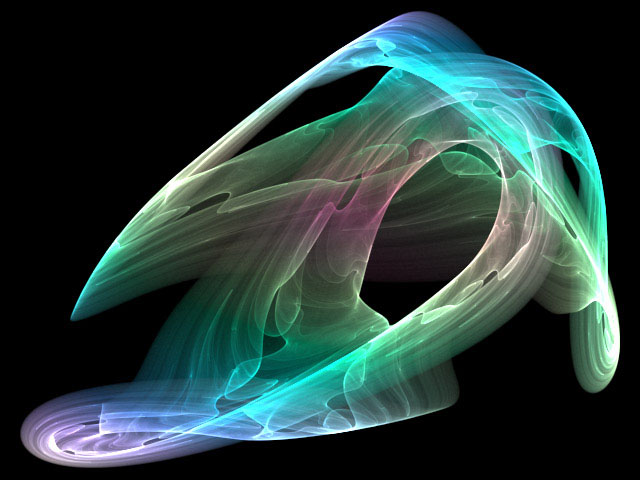## Minoring in MathematicsA mathematics minor makes a great combination with many majors at UGA, including statistics, computer science, biology, engineering, physics, and chemistry.

A minor consists of 15 hours of MATH courses at the 3000 level or above, and must include either

MATH 3200 (Introduction to Higher Mathematics) or
MATH 3500 (Multivariable Mathematics I)

CSCI 2610 can replace MATH 3200 (but only as a prerequisite-- it but does not count towards the "15 hours of MATH courses at the 3000 level or above").

We recommend minor programs such as

MATH MINOR/CS MAJOR:

1. CSCI 2610 (Discrete Mathematics)
2. MATH 3000 (Linear Algebra)
3. MATH 3100 (Sequences and Series)
4. MATH 4670 (Combinatorics)
5. MATH 4500 (Numerical Analysis I)
6. MATH 4510 (Numerical Analysis II)

MATH MINOR/PHYSICS MAJOR

1. MATH 3000 (Linear Algebra)
2. MATH 3200 (Introduction to Higher Mathematics)
3. MATH 3100 (Sequences and Series)
4. MATH 4700 (Ordinary Differential Equations)
5. MATH 4720 (Partial Differential Equations)

Please see the Associate Head of the Department of Mathematics, Dr. Cantarella, Room 451 Boyd Graduate Studies for discussion and approval of your minor program.

Math Minor Completion Form